# Surface integral facts for kids

Kids Encyclopedia Facts

In mathematics, a surface integral is a definite integral taken over a surface (which may be a curve set in space). Just as a line integral allows one to integrate over an arbitrary curve (of one dimension), a surface integral can be thought of as a double integral integrating over a two-dimensional surface. Given a surface, one may integrate over its scalar fields (that is, functions which return numbers as values), or its vector fields (that is, functions which return vectors as values).

Surface integrals have applications in physics, particularly with the classical theory of electromagnetism.The definition of surface integral relies on splitting the surface into small surface elements.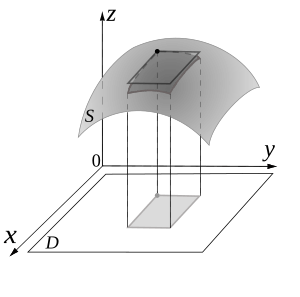An illustration of a single surface element. These elements are made infinitesimally small, by the limiting process, so as to approximate the surface.

## Surface integrals of scalar fields

Consider a surface S on which a scalar field f is defined. If one thinks of S as made of some material, and for each x in S the number f(x) is the density of material at x, then the surface integral of f over S is the mass per unit thickness of S. (This is only true if the surface is an infinitesimally thin shell.)

One approach to calculating the surface integral is then to split the surface in many very small pieces, assume that the density is approximately constant on each piece, find the mass per unit thickness of each piece (by multiplying the density of the piece by its area), and then sum up the resulting numbers to find the total mass per unit thickness of S.

To find an explicit formula for the surface integral, mathematicians parametrize S by considering on S a system of curvilinear coordinates, like the latitude and longitude on a sphere. Let such a parametrization be x(s, t), where (s, t) varies in some region T in the plane. Then, the surface integral is given by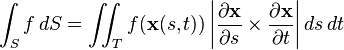$\int_{S} f \,dS = \iint_{T} f(\mathbf{x}(s, t)) \left|{\partial \mathbf{x} \over \partial s}\times {\partial \mathbf{x} \over \partial t}\right| ds\, dt$

where the expression between bars on the right-hand side is the magnitude of the cross product of the partial derivatives of x(s, t).

For example, to find the surface area of some general functional shape, say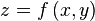$z=f\,(x,y)$, we have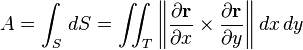$A = \int_S \,dS = \iint_T \left\|{\partial \mathbf{r} \over \partial x}\times {\partial \mathbf{r} \over \partial y}\right\| dx\, dy$

where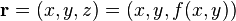$\mathbf{r}=(x, y, z)=(x, y, f(x,y))$. So that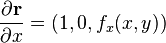${\partial \mathbf{r} \over \partial x}=(1, 0, f_x(x,y))$, and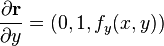${\partial \mathbf{r} \over \partial y}=(0, 1, f_y(x,y))$. So,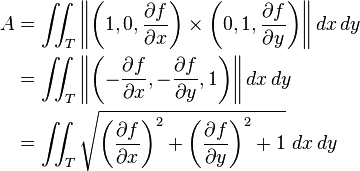\begin{align} A &{} = \iint_T \left\|\left(1, 0, {\partial f \over \partial x}\right)\times \left(0, 1, {\partial f \over \partial y}\right)\right\| dx\, dy \\ &{} = \iint_T \left\|\left(-{\partial f \over \partial x}, -{\partial f \over \partial y}, 1\right)\right\| dx\, dy \\ &{} = \iint_T \sqrt{\left({\partial f \over \partial x}\right)^2+\left({\partial f \over \partial y}\right)^2+1}\, \, dx\, dy \end{align}

which is the formula used for the surface area of a general functional shape. One can recognize the vector in the second line above as the normal vector to the surface.

Note that because of the presence of the cross product, the above formulas only work for surfaces embedded in three dimensional space.

## Surface integrals of vector fields

Consider a vector field v on S, that is, for each x in S, v(x) is a vector.

The surface integral can be defined component-wise according to the definition of the surface integral of a scalar field; the result is a vector. For example, this applies to the electric field at some fixed point due to an electrically charged surface, or the gravity at some fixed point due to a sheet of material. It can also calculate the magnetic flux through a surface.

Alternatively, mathematicians can integrate the normal component of the vector field; the result is a scalar. An example is a fluid flowing through S, such that v(x) determines the velocity of the fluid at x. The flux is defined as the quantity of fluid flowing through S in a unit amount of time.

This illustration implies that if the vector field is tangent to S at each point, then the flux is zero, because the fluid just flows in parallel to S, and neither in nor out. This also implies that if v does not just flow along S, that is, if v has both a tangential and a normal component, then only the normal component contributes to the flux. Based on this reasoning, to find the flux, we need to take the dot product of v with the unit surface normal to S at each point, which will give us a scalar field, and integrate the obtained field as above. This gives the formula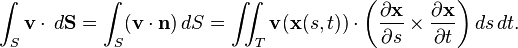$\int_S {\mathbf v}\cdot \,d{\mathbf {S}} = \int_S ({\mathbf v}\cdot {\mathbf n})\,dS=\iint_T {\mathbf v}(\mathbf{x}(s, t))\cdot \left({\partial \mathbf{x} \over \partial s}\times {\partial \mathbf{x} \over \partial t}\right) ds\, dt.$

The cross product on the right-hand side of this expression is a surface normal determined by the parametrization.

This formula defines the integral on the left (note the dot and the vector notation for the surface element).

## Theorems involving surface integrals

Various useful results for surface integrals can be derived using differential geometry and vector calculus, such as the divergence theorem, and its generalization, Stokes' theorem.

### Changing parametrization

The discussion above defined the surface integral by using a parametrization of the surface S. A given surface might have several parametrizations. For example, when the locations of the North Pole and South Pole are moved on a sphere, the latitude and longitude change for all the points on the sphere. A natural question is then whether the definition of the surface integral depends on the chosen parametrization. For integrals of scalar fields, the answer to this question is simple: the value of the surface integral will be the same no matter what parametrization one uses.

Integrals of vector fields are more complicated, because the surface normal is involved. Mathematicians have proved that given two parametrizations of the same surface, whose surface normals point in the same direction, both parametrizations give the same value for the surface integral. If, however, the normals for these parametrizations point in opposite directions, the value of the surface integral obtained using one parametrization is the negative of the one obtained via the other parametrization. It follows that given a surface, we do not need to stick to any unique parametrization; but, when integrating vector fields, we do need to decide in advance which direction the normal will point to, and then choose any parametrization consistent with that direction.

### Parameterizations work on parts of the surface

Another issue is that sometimes surfaces do not have parametrizations which cover the whole surface; this is true for example for the surface of a cylinder (of finite height). The obvious solution is then to split that surface in several pieces, calculate the surface integral on each piece, and then add them all up. This is indeed how things work, but when integrating vector fields, one needs to again be careful in choosing the normal-pointing vector for each piece of the surface, so that when the pieces are put back together, the results are consistent. For the cylinder, this means that if we decide that for the side region the normal will point out of the body, then for the top and bottom circular parts, the normal must point out of the body too.

### Inconsistent surface normals

Last, there are surfaces which do not have a surface normal at each point with consistent results (for example, the Möbius strip). If such a surface is split into pieces, on each piece a parametrization and corresponding surface normal is chosen, and the pieces are put back together, the normal vectors coming from different pieces cannot be reconciled. This means that at some junction between two pieces will have normal vectors pointing in opposite directions. Such a surface is called non-orientable. Vector fields cannot be integrated on non-orientable surfaces.

## Related pagesSurface integral Facts for Kids. Kiddle Encyclopedia.